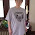## Monday, June 17, 2019

### 911 dialing code #811

Sun, 11 August 1991 = 1st of Elul, 5751
א׳ בֶּאֱלוּל תשנ״א
Parashat Shoftim (in Diaspora)
Rosh Chodesh Elul
"א׳ בֶּאֱלוּל תשנ״א" = 29 (Hebrew Reduction)
"א׳ בֶּאֱלוּל תשנ״א" = 92 (Hebrew Ordinal)
"א׳ בֶּאֱלוּל תשנ״א" = 821 (Hebrew Gematria)142nd prime

911 dialing code #811

From and including: Sunday, August 11, 1991
To, but not including Sunday, August 11, 2019
28 years
"Tishabav" = 28 (Full Reduction)

"Schaumburg, Illinois" = 212 (English Ordinal)
"42° 1′ 49″ N, 88° 5′ 2″ W" = 53 (Full Reduction)

http://www.thepeoplehistory.com/august11th.html

1.Part One of Strange things in gematria
From Thu Oct 24 1985 to Sun Jun 23 2019 is:
- 33 Years, 34 Weeks, 4 Days or 33-344

STONEHENGE AND THE GREAT PYRAMID

The list is impressive, but by no means exhaustive.
The number 37 is a prime - that is, it is only divisible by 1.
How interesting to note that each of the digits, when raised to its triplet, its highest concept, is divisible by 37 - and this in increments of 3, thus:

- 1×37 = 37
- 3×37 = 111
- 6×37 = 222
- 9×37 = 333
- 12×37 = 444
- 15×37 = 555
- 18×37 = 666
- 21×37 = 777
- 24×37 = 888
- 27×37 = 999

136 = Isaiah 41:4
136 = Psalm 118:16

As already noted in chapter 4, the cubic volume of the Great Pyramid is 37,000,000 cubic feet. The mean of earth's orbit around the sun is 186 million miles in diameter, making a circumference of 584.336 million miles. If this distance were stated in inches, it would be 37 with many zeros after it.

The relationship of the number 37 to 27 is of utmost significance because it has to do with the beginning of things, with the sun, with light and with the Elohim. The speed of light is 186,000 miles per second. If this were measured by the reed it would be 93,000.
If 93 were divided by the common denominator of 27, the result would be 3.44 (93÷27 = 3.44), and the number 344 is the gematria for "the light of the world," το φως του κόσμου, (Matt. 5:14). Its connection with the Pyramid is in the gematria for "great wonder," μεγάλο θαύμα, 344. If 344 were the diameter of a circle, the circumference would be 1080 - a number full of meaning.

1.2.Part Two of Strange things in gematria

https://www.ouest-lareunion.com/img-pages/agenda/vignette/agenda_8308.jpg

Grand Boucan = 46, 33, 116
Grand Boucan 2019 = 112, 74, 51, 107, 440
Two Thousand Nineteen = 84, 246, 324
Grand Boucan Two Thousand Nineteen = 923, 109, 1089 (square root 33), 221
Jun 23 (6/23 or 623 or 923) = 76, 118
June Twenty third = 72, 216
Twenty third of June = 84, 222
Sunday Jun 23 2019 = 47, 68, 122
Dreamcatcher = 99, 46, 117
Dragon = 117, 811
Blue dragon = 46, 115
Samurai = 33, 411
Red samurai = 322, 511, 46
Demon = 51, 39
Black demon = 44, 55, 118
Devil = 19, 47
Black devil = 81, 111

Bat = 22, 23, 116
Apple = 23, 22, 47
tentacle = 22, 46, 112

1.